• ## Python文本词频统计

千次阅读 2018-04-09 18:59:35
背景信息本文实现英文文本词频统计功能与Mapreduce中的wordcount是类似的。本文主要是为之后词向量训练做准备工作。本地实现词频统计函数，输入一个文本文件，最终以dict的形式返回词频统计信息。说明：如果输入为...
背景信息本文实现英文文本词频统计，功能与Mapreduce中的wordcount是类似的。本文主要是为之后词向量训练做准备工作。本地实现词频统计函数，输入一个文本文件，最终以dict的形式返回词频统计信息。说明：如果输入为英文文本，则可以直接处理；如果是中文文本，则需要先进行分词等预处理工作。详细代码def word_count(file_name):
import collections
word_freq = collections.defaultdict(int)
with open(file_name) as f:
for l in f:
for w in l.strip().split():
word_freq[w] += 1
return word_freq
#return word_freq.items()   该语句返回值的类型为liststrip()删除空白符（包括'/n', '/r','/t'）；split()以空格分割字符串输入参数——file_name: 表示输入文件名，类型String返回值：返回值为dict，注释行则可以返回list类型的值。测试示例如下示例在Jupyter Notebook中实现，输入文件为《教父》英文小说，通过上述函数统计出每个单词出现的概率。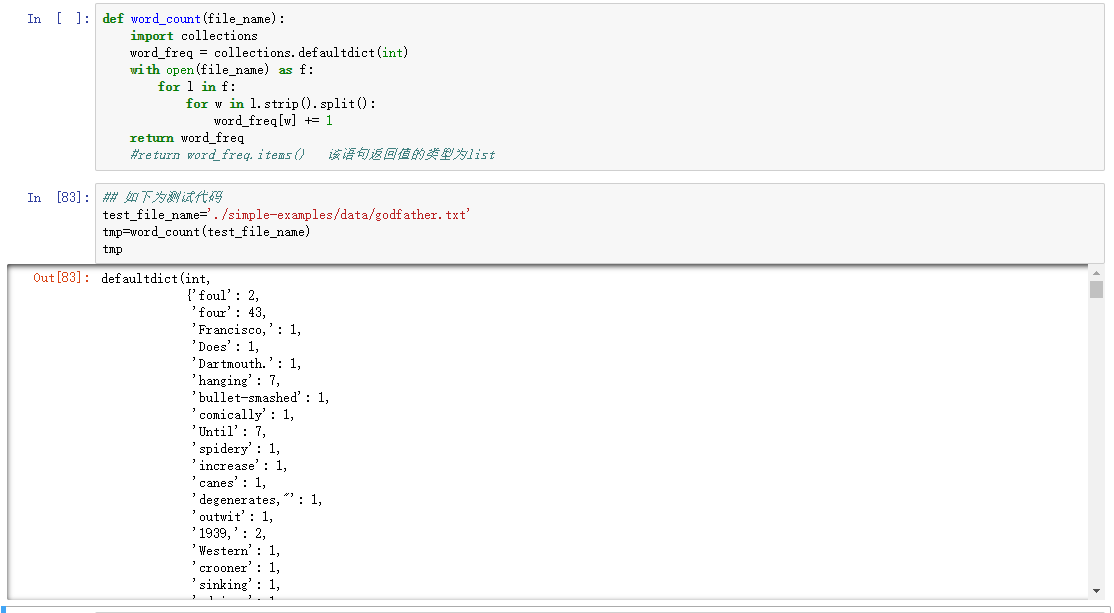展开全文Python
• 中文文本文件之词频统计
文章目录【C语言】中文文本文件之词频统计一、前言二、 代码实现一2.1 源码2.2 执行结果三、代码实现二3.1 源码3.2 执行结果
【C语言】中文文本文件之词频统计
一、前言
以下代码都是针对于小文本文件，不适用于大文本文件
二、 代码实现一
2.1 源码
#include <stdio.h>
#include <stdlib.h>
#include <string.h>

struct Word //声明一个结构体，分别存储单词和对应单词的个数
{
size_t time;
char word;
};

void Copy(struct Word *array, FILE *read, const int length);
void Count_for_word(struct Word *array, const int length);

// 函数一
void Copy(struct Word *array, FILE *read, const int length) //该函数的作用是把文本中的单词复制到数组中
{

char ch, word;
int i = 0, j;

while (fscanf(read, "%s", &word) != EOF)
{
strcpy(array[i].word, word); // 将word复制到arra[i]中
++i;                         //移动数组的下标
}
Count_for_word(array, length); // 调用自定义函数
}

// 函数二
void Count_for_word(struct Word *array, const int length) //统计单词的个数
{
int i, j;

for (i = 0; i < length; i++)
{
array[i].time = 1;
for (j = i + 1; j < length; j++)
{
if (strcmp(array[i].word, array[j].word) == 0)
{
++array[i].time;            //如果遇到相同的单词，就把相应的结构体部分增加 1
strcpy(array[j].word, " "); //并把该单词置为空，因为已经读取到数组中了，所以这里改变的是数组的数据，不影响文本数据
}
}
}

printf("the file have %d word\n\n", length);

for (int index = 0; index < length; index++) // 冒泡排序
{
for (int temp = 0; temp < length - index-1; temp++)
{
// 例如：length = 5
if (array[temp].time < array[temp + 1].time)
{
struct Word word = array[temp];
array[temp] = array[temp + 1];
array[temp + 1] = word;
}
}
}

for (i = 0; i < length; i++)
if (strcmp(array[i].word, " ") != 0)
{ // 当不相等时候
//printf("%-5s occurrs  %-3d %s\n", array[i].word, array[i].time, ((array[i].time > 1) ? "times" : "time"));
printf("%-5s:%-3d\n", array[i].word, array[i].time);
}
}

int main(int argc, char *argv[])
{
char word;
int length = 0, ch;
struct Word *array;

if (argc < 2 || argc > 2)
{
printf("usage: %s filename\n", argv);
exit(EXIT_FAILURE);
}
// 打开文本
if ((read = fopen(argv, "r")) == NULL)
{
printf("open file failure\n");
exit(EXIT_FAILURE);
}
//
while (fscanf(read, "%s", &word) != EOF) //测试是否读到文件末尾
{
++length; //统计文本中单词的个数
}

array = malloc(sizeof(struct Word) * length); // 单词的长度动态分配内存

return 0;
}


2.2 执行结果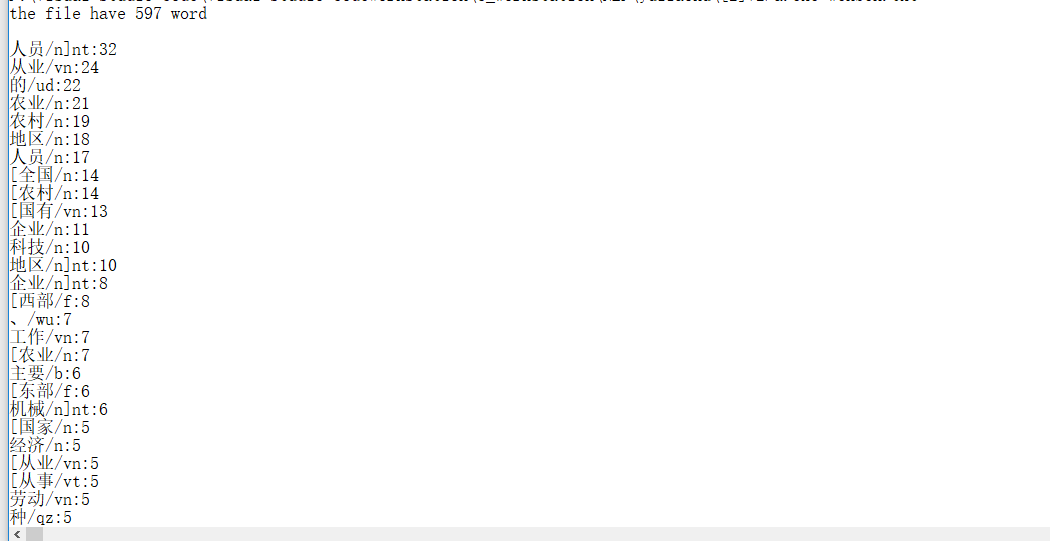三、代码实现二
3.1 源码
#include <stdio.h>
#include <string.h>

struct words //单词结构体
{
char word;
int count;
};

void quick_sort(char s[], int l, int r)
{
if (l < r)
{
int i = l, j = r, x = s[l];
while (i < j)
{
while (i < j && s[j] >= x) //从右到左找到第一个小于x的数
j--;
if (i < j)
s[i++] = s[j];

while (i < j && s[i] <= x) //从左往右找到第一个大于x的数
i++;
if (i < j)
s[j--] = s[i];
}

s[i] = x;                //i = j的时候，将x填入中间位置
quick_sort(s, l, i - 1); //递归调用
quick_sort(s, i + 1, r);
}
}

int main(int argc, char *argv[])
{
struct words word = {0}, stmp = {0};
int i = 0, j = 0, k = 0, flag = 0;
int wors = 0;
char c;
char tmp = {0}; // 存储每个词语，最多存储50个字
FILE *fp = NULL;

fp = fopen(argv, "r");
if (fp == NULL)
{
printf("文本打开错误");
return -1;
}

//读文件解析词语
while ((c = fgetc(fp)) != EOF) // 按字符读取
{
//以换行符作为单词标识符。
if (c != '\n')
{
tmp[j++] = c; // 在未遇到词语标识符之前将
//printf("%s\n",tmp);
}
else
{
tmp[j] = '\0'; // 清零操作
j = 0;         // 重置
flag = 0;

//判断单词是否已经记录 如果已有则使用率加1
for (k = 0; k < i; k++)
{
if (strcmp(tmp, word[k].word) == 0) // 判断相等函数
{
word[k].count++; // 统计相同的词语的个数
flag = 1;        // 设置标识符为1
break;
}
}
//没有则存入单词结构体数组保存
if (!flag)
{
strcpy(word[i].word, tmp);
word[i].count++;
i++;
}
memset(tmp, 0, sizeof(tmp));
memset(tmp, '\0', sizeof(tmp));
}
}

fclose(fp);

int length = sizeof(tmp) / sizeof(char); //求数组的长度
quick_sort(tmp, 0, length);
for (int kk = 0; kk < length; kk++)
{
printf("[%s][%d]\n", word[kk].word, word[kk].count);
}

// 输出排序后的所有已记录单词

/* for (k = 0; k < i; k++)
{
// printf("[%s][%d]\n", word[k].word, word[k].count);
printf("[%s]\n", word[k].word);
} */

return 0;
}


3.2 执行结果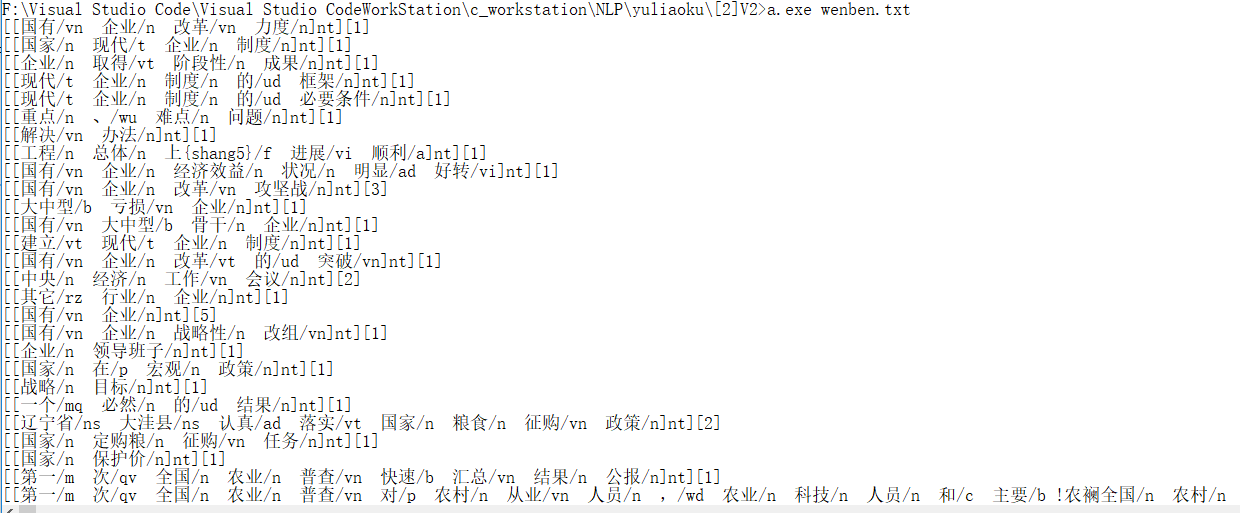展开全文C语言
• 1、文本词频统计 问题需求：一篇文章，出现了哪些词，哪些词出现得最多？ 注意：分英文文本和中文文本以及做好异常处理 # 分析用户给的文本，统计词频并输出词频最高的十个词 import sys import jieba def getText...
组合数据类型练习内容
1、文本词频统计
问题需求：一篇文章，出现了哪些词，哪些词出现得最多？
注意：分英文文本和中文文本以及做好异常处理
# 分析用户给的文本，统计词频并输出词频最高的十个词
import sys
import jieba

def getText(string):
theText = theText.lower()  # 将所有文本变成小写，去除干扰
for ch in '!"#\$%&()*+,-./:;<=>?@[\\]^_‘{|}~':
theText = theText.replace(ch, " ")  # 去除文本中的符号
return theText

print("该程序的功能为分析给定的文本，统计词频并输出词频最高的十个词\n")
choice = input("请选择文本语言:中文(C/c)，英文(E/e)")
counts = {}
try:
if choice not in ['E', 'e', 'C', 'c']:
print("输入有误！")
input("\n按回车键停止程序")
sys.exit(0)
elif choice in ['e', 'E']:
Text = getText(input("请指定文本文件(路径名)："))
words = Text.split()  # 返回一个列表，由空格分隔
for word in words:
counts[word] = counts.get(word, 0) + 1
elif choice in ['c', 'C']:
jieba.setLogLevel(20)  # 去除jieba库的日志统计
words = jieba.lcut(txt)
for word in words:
if len(word) == 1:
continue
else:
counts[word] = counts.get(word, 0) + 1
except FileNotFoundError:
print("文件路径输入有误!!!请检查")
input("\n按回车键停止程序")
sys.exit(0)
items = list(counts.items())
items.sort(key=lambda x: x, reverse=True)
if len(items) < 10:
print("该文本过短，出现词的总个数小于10！")
input("\n按回车键停止程序")
sys.exit(0)
print("\n该文本中出现频率最高的前十个词如下：")
for i in range(10):
word, count = items[i]
print("{:^10}：{:>5}次".format(word, count))
input("\n按回车键停止程序")

2、三国人物出场次数统计
描述：统计并输出三国演义中出场频率前五的人物
# 实现给定"三国演义.txt"文件在工程目录下
import jieba
jieba.setLogLevel(20)  # 去除jieba库的日志统计
excludes = {"将军", "却说", "荆州", "二人", "不可", "不能", "如此"} # 出去非人名词
words = jieba.lcut(txt)
counts = {}
for word in words:
if len(word) == 1:
continue
elif word == "诸葛亮" or word == "孔明曰":
reword = "孔明"
elif word == "关公" or word == "云长":
reword = "关羽"
elif word == "玄德" or word == "玄德曰":
reword = "刘备"
elif word == "孟德" or word == "丞相":
reword = "曹操"
else:
reword = word
counts[reword] = counts.get(reword, 0) + 1
for word in excludes:
del counts[word]
items = list(counts.items())
items.sort(key=lambda x: x, reverse=True)
print("《三国演义》中出场次数前五的人物是：")
for i in range(5):
word, count = items[i]
print("{:^10}：{:>5}次".format(word, count))

运行截图：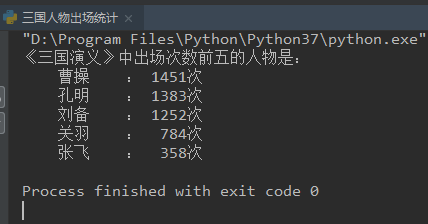3、基本统计值计算
描述：获取以逗号分隔的多个数据输入（输入为一行），计算基本统计值（平均值、标准差、中位数）除中位数外，其他输出保留小数点后两位。
def getNum():  # 获取用户不定长度的输入
s = input()
ls = list(eval(s))
return ls
def mean(numbers):  # 计算平均值
s = 0.0
for num in numbers:
s = s + num
return s / len(numbers)
def dev(numbers, mean):  # 计算标准差
sdev = 0.0
for num in numbers:
sdev = sdev + (num - mean)**2
return pow(sdev / (len(numbers)-1), 0.5)
def median(numbers):  # 计算中位数
numbers.sort()  # 将列表排序
size = len(numbers)
if size % 2 == 0:
med = (numbers[size // 2 - 1] + numbers[size // 2]) / 2
else:
med = numbers[size // 2]
return med
n = getNum()
m = mean(n)
print("平均值:{:.2f},标准差:{:.2f},中位数:{}".format(m, dev(n, m), median(n)))

4、字典翻转输出
描述：
读入一个字典类型的字符串，反转其中键值对输出。
即，读入字典key:value模式，输出value:key模式。
输入格式
用户输入的字典格式的字符串，如果输入不正确，提示：输入错误。
输出格式
给定字典d，按照print(d)方式输出
s = input()
try:
d = eval(s)
e = {}
for k in d:
e[d[k]] = k
print(e)
except:
print("输入错误")



展开全文python 数据分析
• 词频分析是对文章中重要词汇出现的次数进行统计与分析，是文本挖掘的重要手段。它是文献计量学中传统的和具有代表性的一种内容分析方法，基本原理是通过词出现频次多少的变化，来确定...糖果云提供在线词频统计功能

词频分析是对文章中重要词汇出现的次数进行统计与分析，是文本挖掘的重要手段。它是文献计量学中传统的和具有代表性的一种内容分析方法，基本原理是通过词出现频次多少的变化，来确定热点及其变化趋势。

前一阵子有一篇热文，小学生用大数据分析苏轼，说的就是清华附小的学生用词频分析方法对苏轼的作品进行了分析，很多网友解嘲说感觉智商遭到了暴击，其实只要有合适的工具谁都可以进行词频分析，下面介绍一个超强的词频分析网站：糖果云网站 www.tgyun.cc糖果云面向个人及团体提供大数据存储，大数据分析以及大数据交易市场服务。

​糖果云的总体目标是建设一个分类的知识库与数据库，方便全人类的查阅和检索，提高用户对知识获取的速度，提高了工作效率，和学习的进度，为推动人类知识进步做出贡献。

下面我们看看糖果网站提供的词频统计功能，下图是糖果云网站对红楼梦做出的词频统计：

http://www.tgyun.cc/lib/bigtang_红楼梦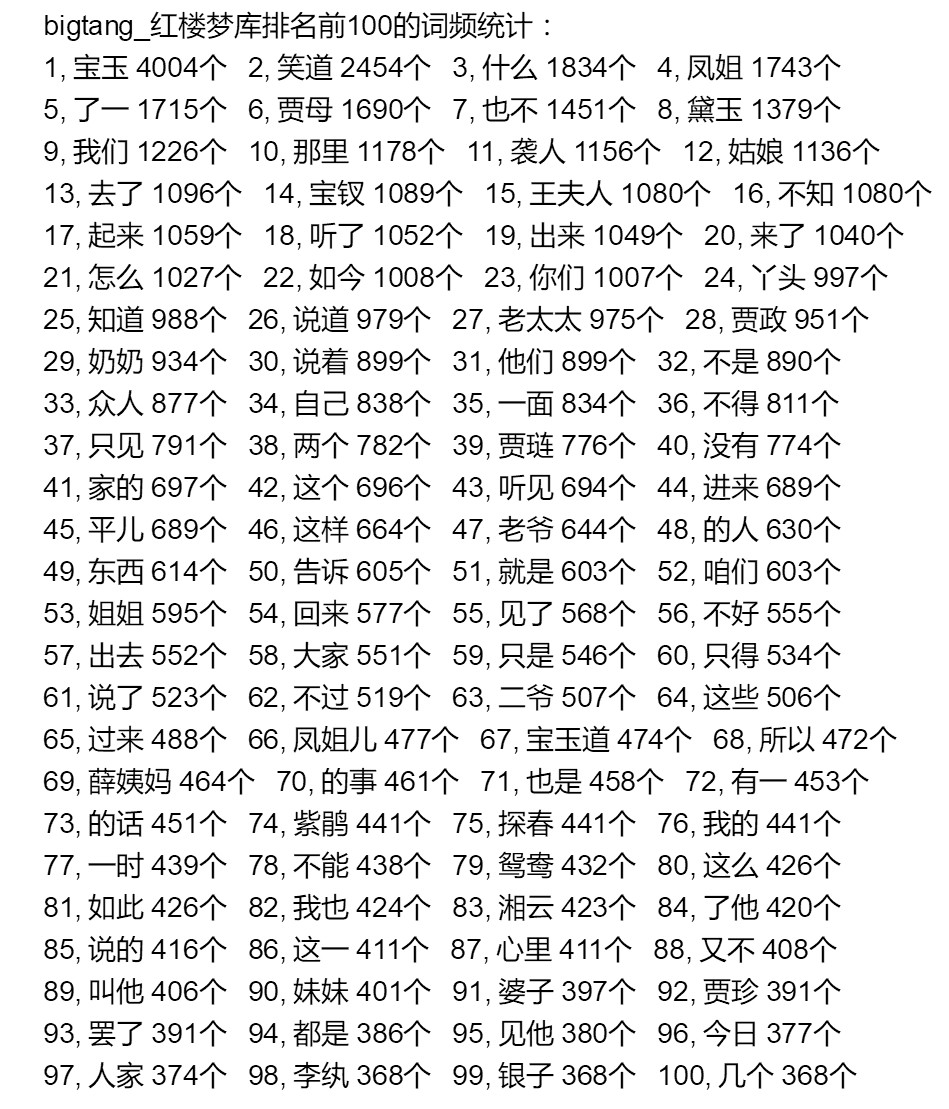从上图可以看到，红楼梦中哪个人物的篇幅最多？哪个人物曹雪芹更注重？这个统计就一清二楚了，我不是红学专家，这个统计里应该还有很多内容可以解说。

说到小学生，就再来看看小学生所做的研究，用大数据来分析苏轼：

下图是糖果云网站对苏轼诗词作品做出的词频统计： http://www.tgyun.cc/lib/user_苏轼诗词全集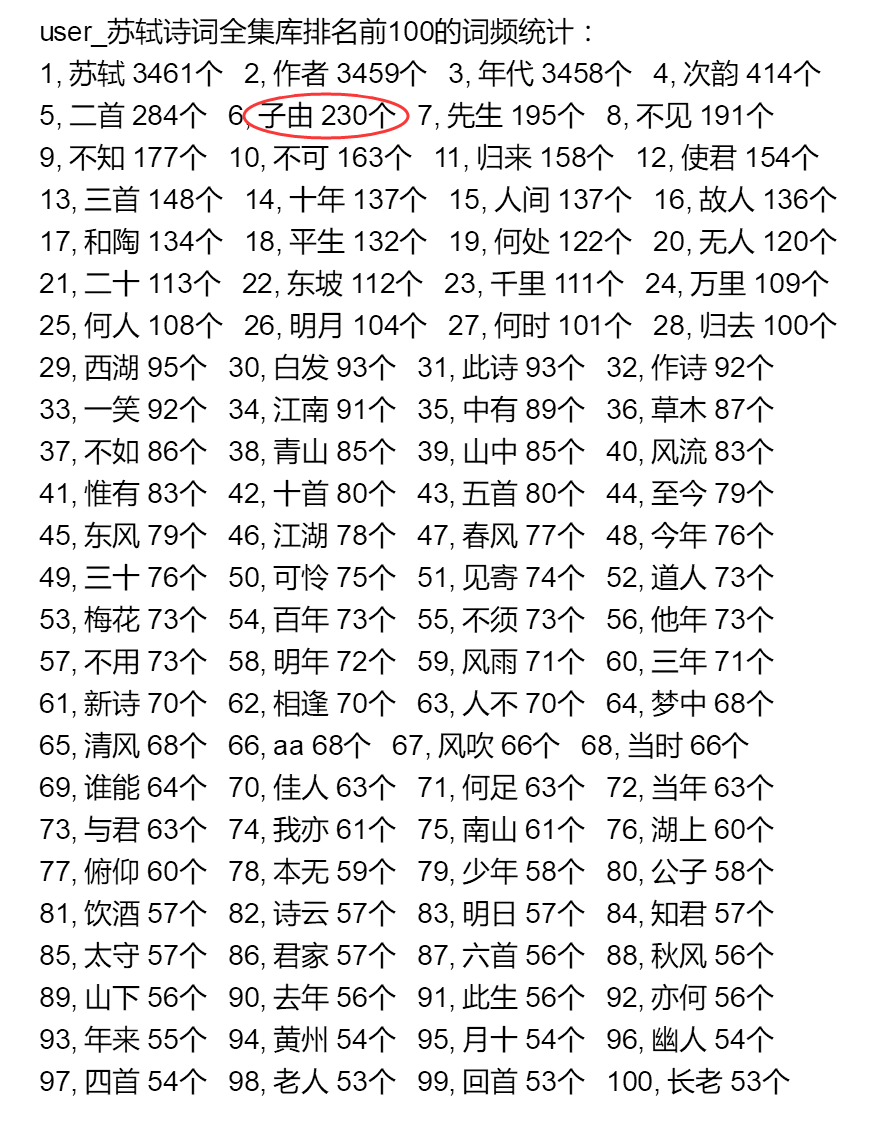词频统计技术涉及到分词技术，分词技术一般有两种做法：

一种是维护一个大词库，文章与词库匹配进行分词，这种方法有个缺点，就是需要不停的加入新词，否则新词统计不到，小学生的分析文章也提到，子由是一个新词，现代汉语没有叫做子由这个词的，那么苏轼的诗词中为什么这么多子由呢？原来子由是苏轼的弟弟！苏轼弟弟苏辙字子由， 苏东波字子瞻。

另一种叫做统计分词，糖果云采用的技术即为统计分词，可以看到子由是个新词，照样被糖果云统计到。这种技术无需担心新词，新词只要有使用量，就可以被统计出来。

糖果云的词频统计超级强大，无论是一篇文章还是几个G的文本，均可轻松统计。
那么怎么对你的文本资料进行词频统计呢？

操作方法：
1 把文章文本资料另存为txt文件；
2 把txt文件压缩成zip文件；
3 把zip文件上传到糖果云，之后，就可以在糖果云库中查找到该文章及词频统计数据了。


展开全文分词 词频分析
• C风格文件、C++风格读写操作 、英文文章单词的正确分割 、基于Trie树实现文件词频统计 、基于Trie树实现带倒排索引的文件词频统计
• 本文实例讲述了Python文本统计功能之西游记用字统计操作。分享给大家供大家参考，具体如下：一、数据xyj.txt，《西游记》的文本，2.2MB致敬吴承恩大师，4020行(段)二、目标统计《西游记》中：1. 共出现了多少个不同...
• 先上个动图，一张图就看明白了。文章目录一．软件使用前的配置：11） 大词典配置：12） 合成音频功能配置：2二.软件功能详细介绍：21）统计翻译22）单词对比43...基本功能： 只需下载软件压缩包（英语词频统计助手....
• C++对中文文本文件进行词频统计及排序欢迎使用Markdown编辑器新的改变功能快捷键合理的创建标题，有助于目录的生成如何改变文本的样式插入链接与图片如何插入一段漂亮的代码片生成一个适合你的列表创建一个表格设定...C++ STL
• 目录 文件和数据格式化 文件的使用 1、概述 2、文件的类型 文件打开和关闭 wordcloud库的使用 1、wordclound库基本介绍 2、wordcloud库使用说明 文本词...
• ## NLPIR（ICTCLAS2016）对词频统计功能

千次阅读 热门讨论 2016-03-16 19:09:29
功能：利用NLPIR（ICTCLAS2016）进行中文分词，并进行词频统计。 备注：win7 64位系统，netbeans编程 代码实现： package cwordseg; import java.io.UnsupportedEncodingException; // import utils.SystemParas; ...ICTCLAS NLPIR java
• word分词中的 org.apdplat.word.WordFrequencyStatistics​ 类提供了词频统计功能 命令行脚本的调用方法如下： 将需要统计词频的文本写入文件：text.txt chmod +x wfs.sh &amp; wfs.sh -textFile=text....word word分词
• 说明：读取单个或多个文本内容(txt,word,pdf),对文章进行分词(中文)，并统计每个词语出现的次数并按从大到小排序。同时通过停用词库排除停用词，并展示结果读取单个文本内容(txt,word,pdf),对文章进行分词(中文)，并...jieba分词 Counter
• 本文主要内容是进行一次中文词频统计。涉及内容包括多种模式下的分词比较和分词词性功能展示。 本次使用的是python的jieba库。该库可在命令提示符下，直接输入pip install jieba进行安装。 Jieba库常用的分词模式...python 自然语言处理
• 中文词频统计 1. 下载一长篇中文小说。 2. 从文件读取待分析文本。 3. 安装并使用jieba进行中文分词。 pip install jieba import jieba ljieba.lcut(text) 4. 更新词库，加入所分析对象的专业词汇。 ...
• 1.下载安装jieba库 利用镜像下载安装 pip install -i https://pypi.tuna.tsinghua.edu.cn/simple jieba 2.jieba库作用与功能概述 jieba库利用中文词库，对中文文本，通过分词，获得单个的词语mysql python 数据分析
• 该段代码能实现抓取网页文本，并进行词频分析的功能。 于是自己对照着一条条敲出来，并且自己添加了注释，最后运行成功时，爽爽滴，看来并不难嘛。代码以下代码实现了抓取一个web页面内容，然后对文本内容进行分词...python
•NLP
• 话说近两年大数据确实火了，带给我们最直接的视觉感受就是利用...核心技术就是：中文分词与词频统计。关于中文分词中文跟英语在分词技术上差别比较多，中文多个字往往可以组成多个词语，而且词语之还可以缩写。例如...
• 上一节 目录~任务说明~要求~进阶~覆盖的知识点（学习）一.自然语言分析的基本术语二. jieba模块学习1.安装jieba模块2.jieba模块常用(1)分词(2)添加自定义字典(3)调整...读取不同格式文本的方法~实操一. txt篇二. do...数据挖掘 大数据
• 功能：实现多级文本预处理全过程，包括文本分词，过滤停用词，词频统计，特征选择，文本表示，并将结果导出为WEKA能够处理的.arff格式。 直接上代码： #!/usr/bin/env python # -*- coding: utf-8 -*- """ 功能...python PyNLPIR
• 笔记来自课程：玩转文本挖掘（51ct0学院），总结整理为个人学习、复习使用，供参考。 对小说第一章进行分词 首先重复之前的操作，读文本数据–章节标识–选取第一章–分词 读取并选取第一章 import pandas as pd ......